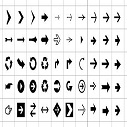# Sort list of dictionaries by values in Python

sorted() function accepts a key function as an argument and calls it on each element prior to make comparison with other elements.

### Using lambda Function

```list_of_dict = [{'ename': 'John', 'salary': 10000},
{'ename': 'Danny', 'salary': 60000},
{'ename': 'John', 'salary': 40000},
{'ename': 'Hanny', 'salary': 30000},
{'ename': 'Korra', 'salary': 50000},
{'ename': 'Aung', 'salary': 40000},
{'ename': 'Marry', 'salary': 60000}]
print(list_of_dict)
print('Sort by salary(Descending)')
print(sorted(list_of_dict, key=lambda x: x['salary'], reverse=True))
print('Sort by Ename(Ascending)')
print(sorted(list_of_dict, key=lambda x: x['ename']))
print('Sort by ename and salary(Ascending)')
print(sorted(list_of_dict, key=lambda x: (x['ename'], x['salary'])))

```

### Using operator.itemgetter

Itemgetter can be used instead of lambda function to achieve the similar functionality. Itemgetter Outputs in the same way as sorted() and lambda does, but has different internal implementation. It takes keys of dictionaries and converts them into tuples. It reduces overhead and is faster and more efficient.

```from operator import itemgetter

print('Using itemgetter:')
print('Sort by salary(Descending)')
print(sorted(list_of_dict, key=itemgetter('salary'), reverse=True))
print('Sort by Ename(Ascending)')
print(sorted(list_of_dict, key=itemgetter('ename')))
print('Sort by ename and salary(Ascending)')
print(sorted(list_of_dict, key=itemgetter('ename', 'salary')))
```Prakash on Jul 12, 2020 at 10:07 am

#### Post Comment

##### WEB TECHNOLOGY##### TypeScript Programmning Language##### New HTML5 APIs##### HTML Arrow -> Range: Decimal 8592-8703. Hex 2190-21FF.##### How To Override One CSS Class With Another×